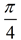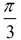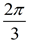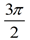Geometry section of MathBitsNotebook.comPractice Test 2 - CCSS Geometry Interactive Version - Multiple ChoiceDirections:  Choose the best answer.  Answer ALL questions (or only a few at a time), then use the BUTTON at the BOTTOM of the page to check your answers.  If you are told that an answer is incorrect, go back and redo that question and CHECK ANSWERS again.   You may CHECK ANSWERS at any time. You may use your graphing calculator when working on these problems.
1. Tad constructs two circles that are tangent to one another. Which of the following statements is always true concerning these circles?
  One circle is in the interior of the other circle.
  The two circles are congruent.
  The two circles are similar.
  The two circles have equal area.

2.
ΔABC is an isosceles triangle with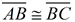. If mA = 3x + 6 and mB = 6x, find mC.
 14º
 48º
 70º
 84º

 3.  Lines m and n are parallel with m∠2 = x² - 10x and m∠1 = 2x + 132. What is the m∠3?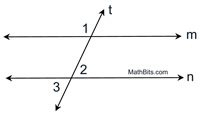12º
 24º
 30º
 40º

4.  A circle with a center at (2,1) passes through the point (5,4). What is the length of the diameter of this circle?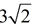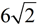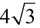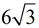5.  Point P is a point located on segment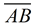such that AP:PB=1:2. If A(-4,-5) and P(-2,-1), find the coordinates of point B.
   (2,7)   (0,3)   (2,4)   (-1,0)

6.
What is the smallest number of degrees by which a regular hexagon may be rotated about its center and appear unchanged?
  180º   72º   60º   45º

7.
Which of the following statements will prove that a quadrilateral is a rhombus?
 The opposite sides are parallel and congruent.
 The diagonals are congruent and perpendicular.
 Both pairs of opposite sides are congruent and the adjacent sides are perpendicular.
 The diagonals are perpendicular and bisect each other.

8.  What is the center, C, and the radius, r, of a circle with the equation x2 - 4x + y2 + 6y = 3 ?
   C (-2,3), r = 4   C (2,-3), r = 16   C (4,-6), r = 3   C (2,-3), r = 4

9.
Which transformation on ΔABC will result in image ΔA'B'C' , as shown on the graph at the right?
   reflection over the y-axis   translation (x, y) → ( x - 4, y - 4)   reflection over the x-axis, followed by T-4,0   rotation of 180º centered at the origin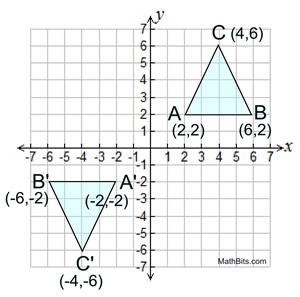10.  Tanya is attempting to inscribe a circle in a triangle by construction. Which construction must she use to locate the center of the circle?
   angle bisectors of two angles   perpendicular bisectors of two sides   altitudes from two vertices of the triangle   two medians of the triangle

11.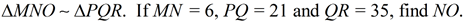3.6   5  10   15

12.  As seen at the right,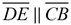and BE = 8. If CD : DA = 2 : 5,
what is AB ?
   10   16   20   28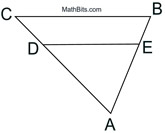13.  In ΔRST, mR = 35º and mS = 65º. Which choice is accurate as to the lengths of the sides of the triangle?
  RS < ST < RT  RS < RT < ST  RT < ST < RS  TS < RT < RS

14 .
Given that sin(2x + 10) = cos(3x + 40), find the number of degrees in the acute angles of the corresponding right triangle.
 20º and 70º
 25º and 65º
 26º and 64º
 30º and 60º

15.  A roof is modeled by ΔPQR where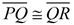and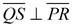. If mQPS = 28º and PR = 28 feet, what is QS, to the nearest tenth of a foot ?
   6.6 feet   7.4 feet   8.1 feet   15.0 feet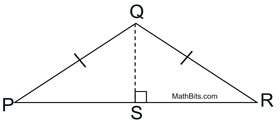m∠RQP = 90º.   ∠QRS and ∠RSP are supplementary angles.   ∠RQP is congruent to ∠RSP.   ∠RQP and ∠RSP are supplementary angles.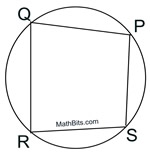17.  Which linear equation is not parallel to the other linear equations listed?
  3y - 2x + 5 = 0   y = (2/3)x + 6  9y - 6x = -3  2y - 3x - 2 = 0

18.  In isosceles ΔABC,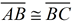and median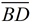is drawn. Prior to proving thatis also an altitude, it will be necessary to prove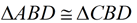by _____.
  Hypotenuse Leg   Side-Side-Side   Angle-Side-Angle   Angle-Angle-Side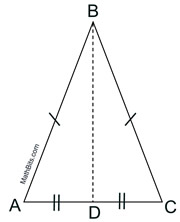19.
A cylinder has a radius of 6 and a height of 12. A cone has the same radius, but need to have the same volume as the cylinder. What must be the height of the cone so its volume will be the same as the volume of the cylinder?
 6
 12
 24
 36

20.
In circle O, AP =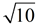, PB = x and BC = 3.
Find the value of x.
   5    2   3    4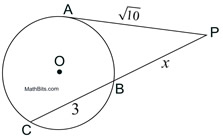21.
Which of the following triangles are always similar?
  Equilateral triangles   Isosceles triangles  Obtuse triangles   Scalene triangles

22.
In the diagram at the right,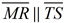,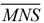and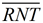.
What is the value of x ?
   4    8   10    12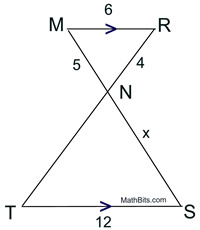23.  In circle O, the arcs are in the ratio EF:FG:GH:HE = 2 : 5 : 4 : 7.
What is the mEMF ?
   20º   40º   60º    80º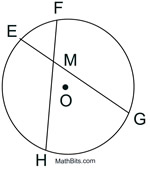24.  What is the radian measure of the smaller angle formed by the hands of a clock at 4 pm?.